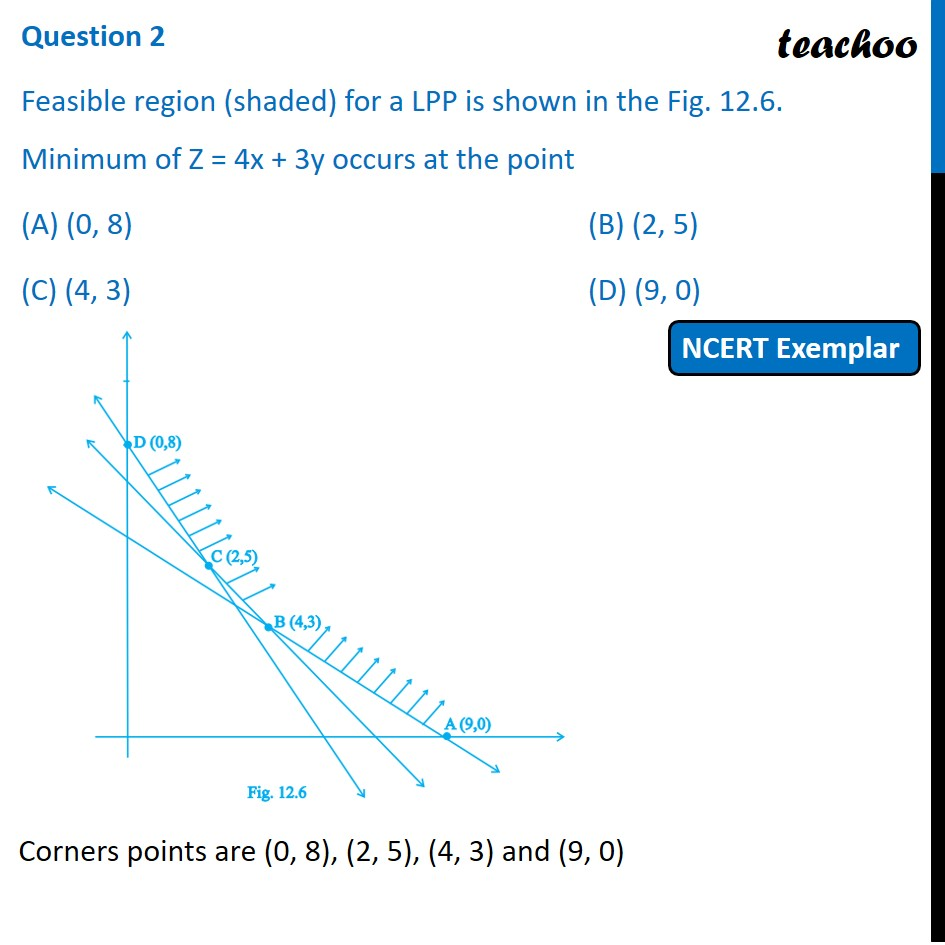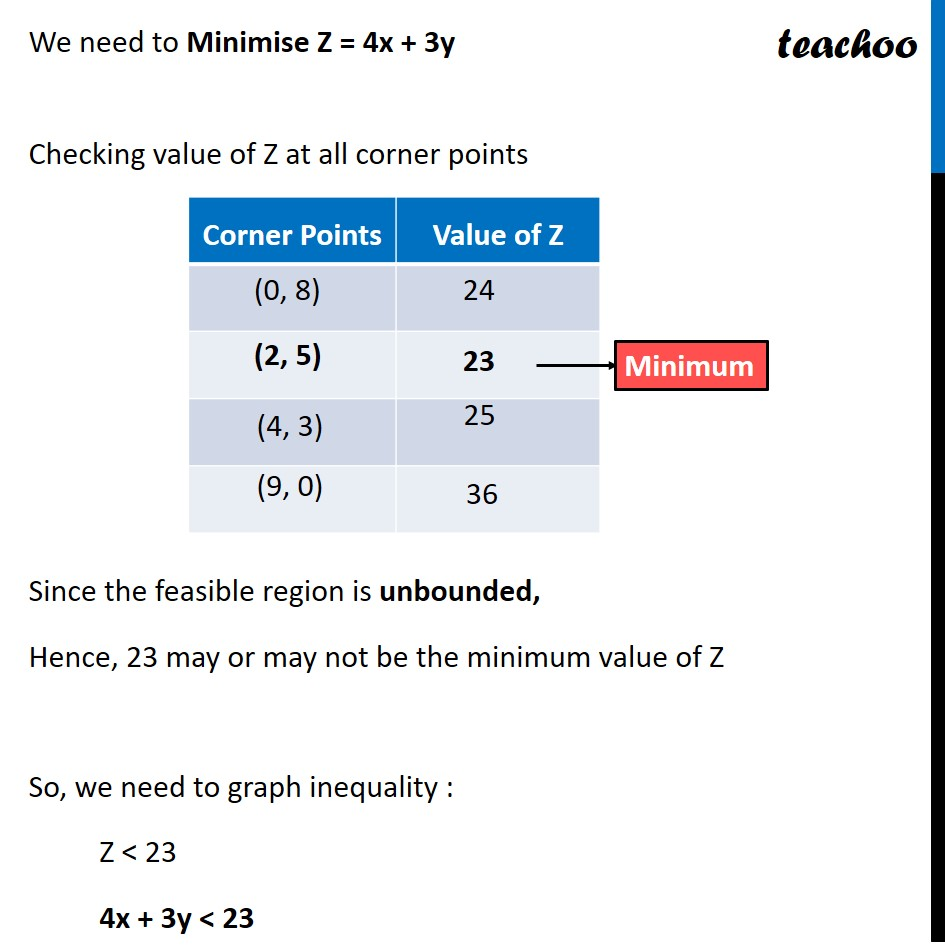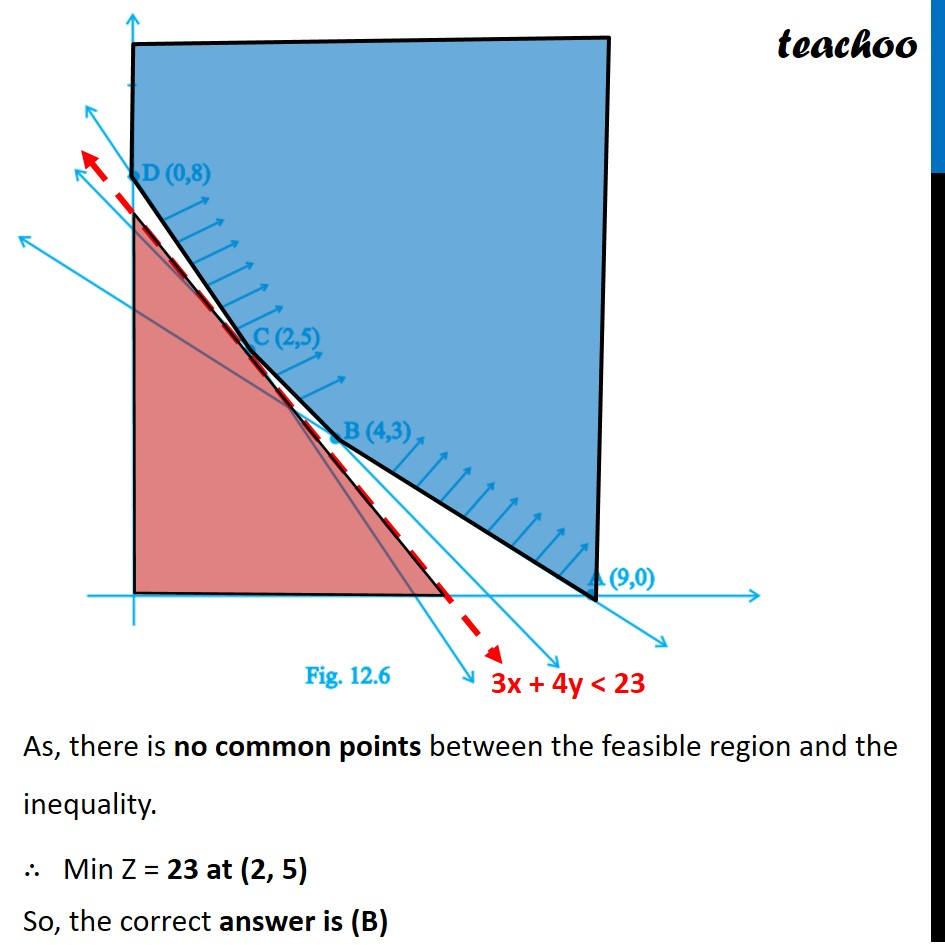NCERT Exemplar - MCQs

Chapter 12 Class 12 Linear Programming
Serial order wise

## (C) (4, 3)                           (D) (9, 0)

This question is similar to Ex 12.2, 1 - Chapter 12 Class 12 - Linear ProgrammingLearn in your speed, with individual attention - Teachoo Maths 1-on-1 Class

### Transcript

Question 2 Feasible region (shaded) for a LPP is shown in the Fig. 12.6. Minimum of Z = 4x + 3y occurs at the point (A) (0, 8) (B) (2, 5) (C) (4, 3) (D) (9, 0) Corners points are (0, 8), (2, 5), (4, 3) and (9, 0) We need to Minimise Z = 4x + 3y Checking value of Z at all corner points Since the feasible region is unbounded, Hence, 23 may or may not be the minimum value of Z So, we need to graph inequality : Z < 23 4x + 3y < 23 As, there is no common points between the feasible region and the inequality. ∴ Min Z = 23 at (2, 5) So, the correct answer is (B)